# Young diagram

(diff) ← Older revision | Latest revision (diff) | Newer revision → (diff)

of orderA graphical representation of a partitionof a natural number(where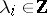,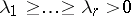,). The Young diagramconsists ofcells, arranged in rows and columns in such a way that the-th row has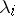cells, where the first cell in each row lies in one (the first) column. E.g., the partition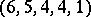of 20 is represented by the Young diagram (cf. the diagram on the left).The transposed Young diagramcorresponds to the conjugate partition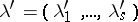, whereis the number of cells in the-th column of the Young diagram. Thus, in the example given above the conjugate partition will be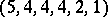.

Each cell of a Young diagram defines two sets of cells, known as a hook and a skew-hook. Let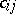be the cell situated in the-th row and the-th column of a given Young diagram. The hook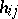corresponding to it is the set consisting of all cells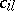,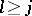, and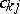,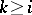, while the skew-hook is the least connected set of border cells including the last cell of the-th row and the last cell of the-th column. E.g. for the Young diagram chosen on the left, the hook and skew-hook corresponding to the cellhave the shape shown in the centre and on the right of the figure.

The length of a hook (respectively, a skew-hook) is understood to be the number of its cells. The length of the hookis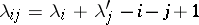. By removing from a Young diagram a skew-hook of lengthone obtains a Young diagram of order. The height of a hook (respectively, a skew-hook) is understood to be the number of rows over which the hook (skew-hook) is distributed.

The language of Young diagrams and Young tableaux (cf. Young tableau) is applied in the representation of the symmetric groups and in the representation of the classical groups. It was proposed by A. Young (cf. ).

How to Cite This Entry:
Young diagram. Encyclopedia of Mathematics. URL: http://encyclopediaofmath.org/index.php?title=Young_diagram&oldid=11674
This article was adapted from an original article by E.B. Vinberg (originator), which appeared in Encyclopedia of Mathematics - ISBN 1402006098. See original article